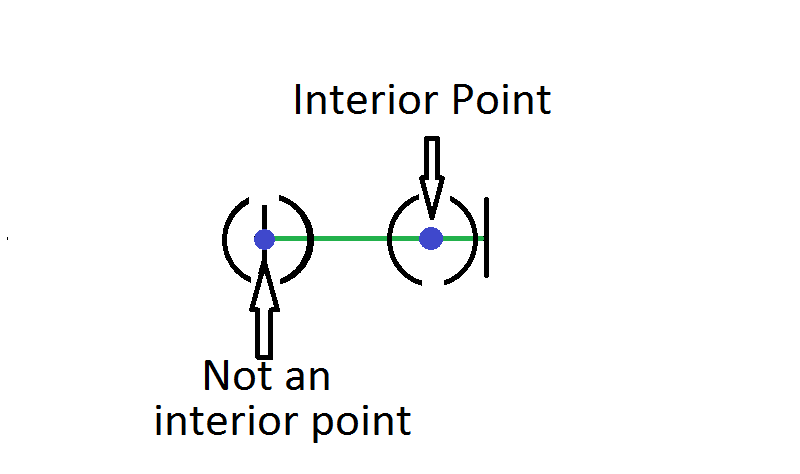Geometry

# Analysis Warmup

Which of these is the set of all interior points of the open interval $(0,1)?$

Definition. An interior point of a set $S$ of real numbers is a number $x$ such that the open interval $(x - \epsilon, x + \epsilon)$ is contained in $S$ for some positive number $\epsilon.$

Which of these is the set of all boundary points of the closed interval $[0,1]$?

Definition. A boundary point of a set $S$ of real numbers is a number $x$ such that every open interval $(a, b)$ contaning $x$ contains a number in $S$ and a number not in $S.$

Which of these is the set of all interior points of the closed interval $[0,1]?$

Definition. An interior point of a set $S$ of real numbers is a number $x$ such that the open interval $(x - \epsilon, x + \epsilon)$ is contained in $S$ for some positive number $\epsilon.$True or False?

The set of all boundary points (using the definition below) of the natural numbers $\mathbb{N}$ is $\mathbb{N}.$

Definition. A boundary point of a set $S$ of real numbers is a number $x$ such that every open interval $(a, b)$ containing $x$ contains a number in $S$ and a number not in $S.$

What is

$\bigcup_{n=3}^\infty \left[\frac{1}{n},1 - \frac{1}{n}\right]?$

Notation. The bracket notation represents a closed interval, so $[3, 5] = \{x | 3 \leq x \leq 5\}$ is the set of all real numbers greater than or equal to 3 and less than or equal to 5.

The $\bigcup$ symbol represents set union, so $\{1, 2, 3\}\bigcup \{2,3,4\} = \{1, 2, 3, 4\}.$ The indexing $\bigcup_{n=3}^\infty$ represents an union of infinitely many sets, i.e.

$\bigcup_{n=3}^\infty \left[\frac{1}{n},1 - \frac{1}{n}\right] = \left[\frac{1}{3},1-\frac{1}{3}\right] \bigcup \left[\frac{1}{4},1- \frac{1}{4}\right] \bigcup \left[\frac{1}{5},1- \frac{1}{5}\right] \bigcup \cdots$

×